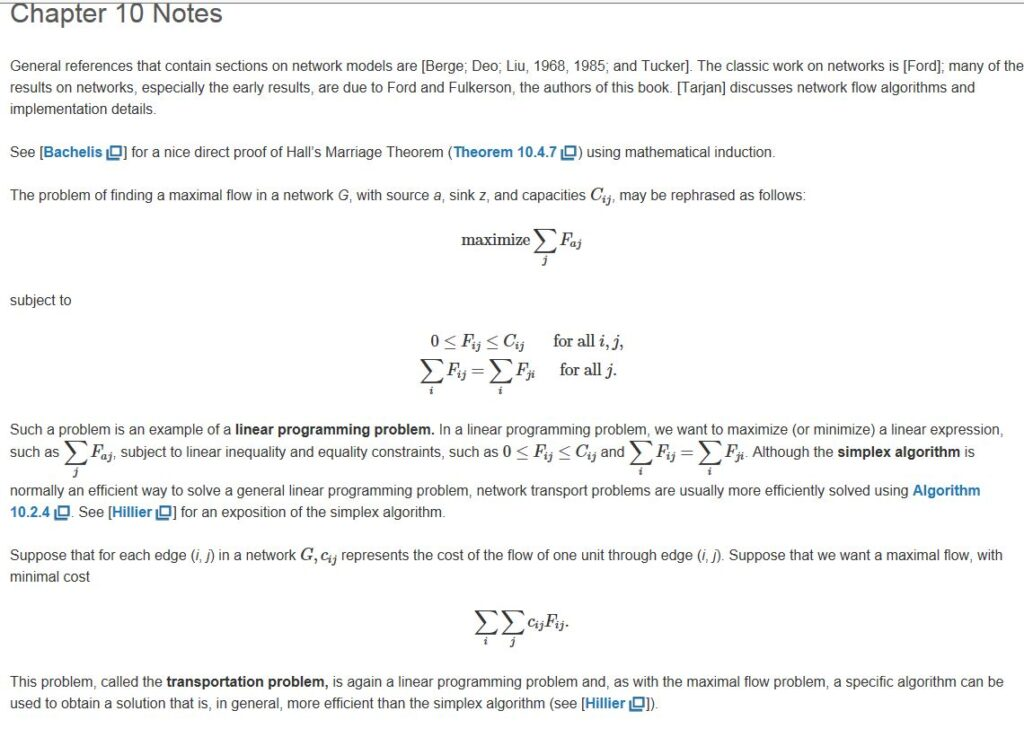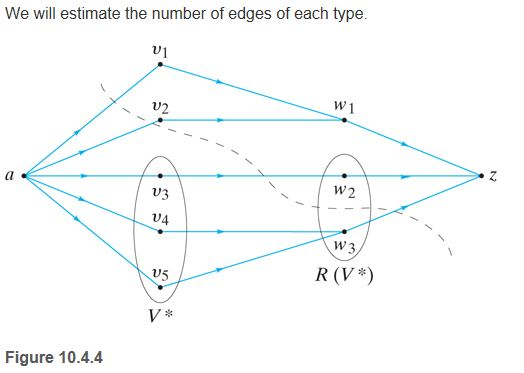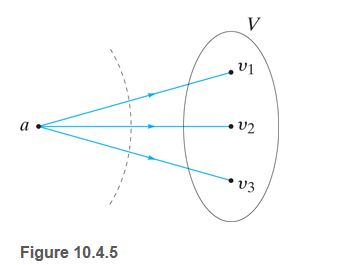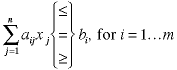# Discrete Math and Computer Science Optimization Problem Solution Sample

QUESTION

In the past few units, you have learned about many discrete math and computer science optimization problems, including min cut, max flow; shortest paths; minimum spanning trees; and matching. Each of these optimization problems can be rephrased or rewritten as an equivalent linear programming problem. A linear programming problem consists of an objective (that is, a value to be maximized or minimized) and constraints.

Each of the graphical optimization problems discussed in this class can be rephrased as a linear programming problem by altering the objective or the constraints to refit the problem at hand. Another way to use a linear program to solve an optimization problem is to transform a new problem into a problem for which we already have a linear program solution—this is a reduction. The idea is that you already have a solution for a known linear programming problem. If you can somehow transform a new problem that you have into this known linear programming problem, you already have the solution. The tricky part is to figure out how to transform your problem into a known solution problem.

For this assignment, read the Notes section on page 529 of your textbook Screenshot at the bottom of the problem. There is a linear program on this page that describes finding a maximal flow in a network G, with a source a, a sink z, flows F, and capacities C. If we want to find a solution to a different problem, maybe the matching problem, for example, we could (1) formulate a linear program for the matching problem or (2) transform the matching problem into a maximal flow problem, then use the already known linear program that solves the maximal flow problem.

To better understand linear programs and reductions, write a two- to three-page paper that addresses the following questions:

1. What is a linear program? Explain in at least one paragraph. Feel free to show an example.
2. What are constraints in linear programming problems? Offer at least one example.
3. What is an optimization problem? Offer an example and explain.
4. What is a reduction (specifically, changing one problem into another)? Provide an example. You may use the Internet to locate an example.
5. Given Example 10.4.4 and Theorem 10.4.5 in the textbook Examples at the bottom of this problem, explain how you would transform the matching problem into a maximal flow problem.
6. Once you complete question 5, use the already known linear program that solves the maximal flow problem. Show all of your work and how you are doing the reduction.

Show all of your work and include references for resources you use to complete the assignment.

Hint 1: Transform the matching problem into a matching network, and then find the maximal flow to this network.

Hint 2: The goal here is to learn that, while problems might appear different, they are often the same. Knowing how to transform a new problem into a known problem can save a lot of solving time. Use the Internet and your textbook.1. Linear Program :

A linear program is a linear function having some constraints which may be equalities or inequalities. A function F of variables x1,x2,…,xn will be a linear function iff for a set of constants s1,s2,…,sn , F(x1,x2,…,xn) = s1 x1+s2 x2+…sn xn .

For an example, F= 3x1 + 4x2 – x3 .

1. Constraints in Linear Programming Problems:

In linear programming problems, the constraints can be both equalities and inequalities. These constraints define the limitations of the decision. Sources such as contractual obligations, physical laws or limited resources can cause constraints. A linear program can have m constraints that can be shown asFor example

1. x1 + 5x2 ≤ 9
2. -4x1 + 2x2 ≥ 0
3. 3x1 + 6x2 = 8
1. Optimization Problem:

In mathematics, optimization problem is one which focuses on maximization or minimization of mathematical function in a provided region.

For an example,

Let’s suppose a dark chocolate manufacturing company which produces only two types of dark chocolates –X and Y. Both the chocolates require Choco and Milk only.  To manufacture each unit of X and Y, following quantities are required:

• Each unit of X requires 2 unit of Milk and 5 units of Choco
• Each unit of Y requires 2 unit of Milk and 4 units of Choco

The company kitchen has a total of 10 units of Milk and 22 units of Choco. On each sale, the company makes a profit of

• Rs 18 per unit A sold

• Rs 15 per unit B sold.

Now, the company wishes to maximize its profit. Using optimization we can easily find how many units of X and Y should it produce respectively.

1. Reduction:

Reduction is an algorithm for converting one existing problem into another so that the solution of second problem can offer the solution of first problem.

For an example,

Euclidean MST reduces to Voronoi.

To solve an Euclidean MST on N points, we need following algorithm:

• Solve Voronoi for those N points.

• Construct graph with linear number of edges.

• Use Prim’s algorithm to find MST in time proportional to N log N.

References:

1. Columbia.edu. (2019). [online] Available at: http://www.columbia.edu/~cs2035/courses/ieor3608.F04/lpdef.pdf [Accessed 10 Mar. 2019].
2. Analytics, B. and scientists, I. (2019). Introductory guide on Linear Programming explained in simple english. [online] Analytics Vidhya. Available at: https://www.analyticsvidhya.com/blog/2017/02/lintroductory-guide-on-linear-programming-explained-in-simple-english/ [Accessed 10 Mar. 2019].
3. Cs.princeton.edu. (2019). [online] Available at: https://www.cs.princeton.edu/~rs/AlgsDS07/23Reductions.pdf [Accessed 10 Mar. 2019].

Looking for best Mathematics Assignment Help. Whatsapp us at +16469488918 or chat with our chat representative showing on lower right corner or order from here. You can also take help from our Live Assignment helper for any exam or live assignment related assistance